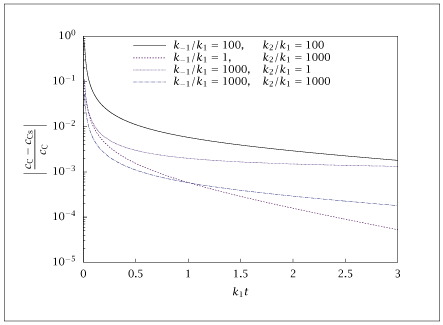## Figure 5.12:

### Fractional error in the QSSA concentration of C versus dimensionless time for the series-parallel reaction, A<-> B-> C.## Code for Figure 5.12

### main.m

```
1
2
3
4
5
6
7
8
9
10
11
12
13
14
15
16
17
18
19
20
21
22
23
24
25
26
27
28
29
30
31
32
33
34
35
36
37
38
39
40
41
42
43
44
45
46
47
48
49
50
51
52
53
54
55
56
57
58
59
60
61
62
63
64
65
66
67
68
69
70
71
72
73
74
75
76
77
78
79
80
81
82
83
84
85
86
87
88
89
90
91
92
93
94
95
96
97
98
99
100% Copyright (C) 2001, James B. Rawlings and John G. Ekerdt
%
% This program is free software; you can redistribute it and/or
% modify it under the terms of the GNU General Public License as
% published by the Free Software Foundation; either version 2, or (at
% your option) any later version.
%
% This program is distributed in the hope that it will be useful, but
% WITHOUT ANY WARRANTY; without even the implied warranty of
% MERCHANTABILITY or FITNESS FOR A PARTICULAR PURPOSE.  See the GNU
% General Public License for more details.
%
% You should have received a copy of the GNU General Public License
% along with this program; see the file COPYING.  If not, write to
% the Free Software Foundation, 59 Temple Place - Suite 330, Boston,
% MA 02111-1307, USA.

% This program "schm2_error.m" generates error curves for the
% reversible series problems as the reverse rate consant and
% the second rate constant are varied.  First-order reactions

% Last edited 1/30/97.

% Revised 7/24/2018

p = struct(); % Create structure to pass parameters to ode15s function
p.k1 = 1.0;

Initial = [1,0,0]';
t = [0:.01:3]';
t_tmp = t(2:length(t));

%
p.k_1 = 100;
p.k2 = 100;
opts = odeset ('AbsTol', sqrt (eps), 'RelTol', sqrt (eps));

[tsolver, solution] = ode15s(@(t,x) rxrate(t,x,p),t,Initial,opts);
alpha = (p.k1*p.k2)/(p.k_1 + p.k2);
c_Css = 1-exp(-alpha*t_tmp);
c_Cexact = solution(2:end,3);
E = (c_Cexact - c_Css)./c_Cexact;
EE = abs(E);
error = log10(EE);
% JBR, 2/22/98

%
p.k_1 = 1;
p.k2 = 1000;

[tsolver, solution] = ode15s(@(t,x) rxrate(t,x,p),t,Initial,opts);
alpha = (p.k1*p.k2)/(p.k_1 + p.k2);
c_Css = 1-exp(-alpha*t_tmp);
c_Cexact = solution(2:end,3);
E = (c_Cexact - c_Css)./c_Cexact;
EE = abs(E);
error = log10(EE);
% JBR, 2/22/98

%
p.k_1 = 1000;
p.k2 = 1;

[tsolver, solution] = ode15s(@(t,x) rxrate(t,x,p),t,Initial,opts);
alpha = (p.k1*p.k2)/(p.k_1 + p.k2);
c_Css = 1-exp(-alpha*t_tmp);
c_Cexact = solution(2:end,3);
E = (c_Cexact - c_Css)./c_Cexact;
EE = abs(E);
error = log10(EE);
% JBR, 2/22/98

%
p.k_1 = 1000;
p.k2 = 1000;

[tsolver, solution] = ode15s(@(t,x) rxrate(t,x,p),t,Initial,opts);
alpha = (p.k1*p.k2)/(p.k_1 + p.k2);
c_Css = 1-exp(-alpha*t_tmp);
c_Cexact = solution(2:end,3);
E = (c_Cexact - c_Css)./c_Cexact;
EE = abs(E);
error = log10(EE);
% JBR, 2/22/98

if (~ strcmp (getenv ('OMIT_PLOTS'), 'true')) % PLOTTING
% TITLE
end % PLOTTING

```

### rxrate.m

```
1
2
3
4
5
6
7
8
9function dcdt = rxrate(t,x,p)
c1 = x(1);
c2 = x(2);
c3 = x(3);

r1 = p.k1*c1 - p.k_1*c2;
r2 = p.k2*c2;

dcdt = [-r1; r1-r2; r2];

```